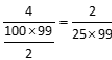# Problem Solving Question: Number Properties

This question combines number properties and probability concepts. The focus is on understanding what kind of numbers have exactly 3 factors and elementary counting methods.

## Question

• PS
• If two distinct integers a and b are picked from {1, 2, 3, 4, .... 100} and multiplied, what is the probability that the resulting number has EXACTLY 3 factors? A.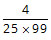B.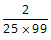C.D.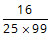E.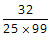Choice B. The required probability isClick to access Explanatory Answer slide deck

## Detailed Solution

What kind of numbers have exactly 3 factors?

Any positive integer will have '1' and the number itself as factors. That makes it a minimum of 2 factors (except '1' which has only one factor). If the positive integer has only one more factor, then in addition to 1 and the number, the square root of the number should be the only other factor.

There are two key points in the above finding. The number has to be a perfect square. And the only factor other than 1 and the number itself should be its square root.

Therefore, if a positive integer has only 3 factors, then it should be a perfect square and it should be the square of a prime number.

Let us look at an example. 4 has the following factors: 1, 2, and 4 (exactly 3 factors). It is the square of '2' which a prime number.

Let us look at one more example. 25 has the following factors: 1, 5, and 25 (exactly 3 factors). It is the square of '5' which is a prime number.

Squares of numbers that are not prime numbers will have more than 3 factors. For instance, 36 is a perfect square. But it has 9 factors.

So, the first step to solving this question is to count the number of squares of prime numbers from 1 to 100
4, 9, 25, and 49 are the 4 numbers that have exactly 3 factors.

The second step is to compute the number of ways of selecting two distinct integers from the set of first 100 positive integers.
Two distinct integers can be selected from the set of 100 positive integers in 100C2 ways.
This value is the denominator of the required probability.

Now, we need to compute the numerator to arrive at the required probability.
We have to compute the number of outcomes in which the product of two numbers will result in a number that has 3 factors
Or, we have to compute the number of outcomes in which the product of the two numbers will be 4 or 9 or 25 or 49.

The product of two numbers 'a' and 'b' will be 4 when one of the numbers is 1 and the other is 4. There is only one set that will result in this product.
The same holds good for the other 3 numbers as well. Product of 'a' and 'b' will be 9 when one of the numbers is 1 and the other is 9 and so on.
Therefore, there are 4 outcomes in which the product of the two numbers will result in a number that has exactly 3 factors.

Hence, the required probability =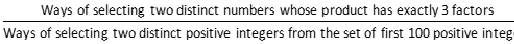.

i.e.,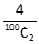Or the required probability =